## Pascal's Trapezoid and Some Puzzles

2017 May 22

Okay, time for me to vomit miscellaneous information about Pascal's Triangle into a text document again.

Find and prove a formula for when it converges.

I recently thought about Pascal's Triangle in a different way and came up with a different way of explaining why its entries are given by the combinations formula. It is somewhat more satisfying than the inductive proof, as it provides a reason for the relationship between the two.

Think of Pascal's Triangle as a kind of game board, with each entry being a space on the board. We start with our game piece on the top square a move consists of the movement of our piece down one level to either the square below it and to the left or below it and to the right, and moving up or directly to either side is not allowed. Now suppose we want to find the number of distinct paths we can take from the starting point to any square on the board. Now consider this. Since each path to some square must pass through one of the squares above it, the number of paths to some square is the sum of the number of paths to each of the squares above it. Because of this property, it is now obvious that the number inside of any square in Pascal's Triangle actually describes the number of such paths.

If we send our piece always to the left, it would always hug the left edge of the pyramid, and each step to the right is a step away to the edge. Let us call the topmost row the 0th row and the leftmost entry in each row the 0th entry. Then a path to any square in the nth row requires $n$ moves, and if an entry is the rth in its row, then its path must have $r$ steps to the right. We can reduce each path to a string of Ls and Rs, since at each step, a path must go either down and left or down and right. Then the string of a path going to the rth square in the nth row must then be a string of length $n$ containing exactly $r$ Rs, and the number of distinct paths is the number of ways to formulate such a string. Since the number of strings in equal to the number of ways to choose $r$ locations in which to place an R in a string of length $n$ otherwise consisting of Ls, by definition, the number of such strings is $_nC_r$, and so the rth entry in Pascal's Triangle in the nth row is given by $_nC_r$.

Using this interpretation of Pascal's Triangle, I can now solve a problem that has puzzled and intrigued me for some time - the problem of finding the formula for any entry in Pascal's Trapezoid (just like the triangle, but starts with a row of ones instead of a single entry that is a one). Here's an example of such a trapezoid: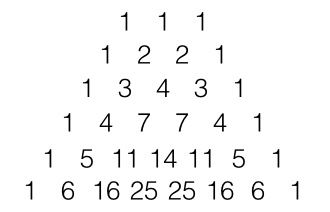If we think of these in the same way that we did with Pascal's Triangle, we can see that each entry will be the number of paths from the top to that entry's square. Since there are multiple starting places, the number of paths to some square is the sum of the paths to that square from each of the squares on the top row.

Again, let use call the top row the 0th row and the leftmost entry in each row the 0th entry. Then the number of paths from the 0th starting point is $nC_r$, the number of paths from the 1st starting point is $_nC{r-1}$, and the number from the 2nd is $nC{r-2}$. Thus the rth entry in the nth row is

Without loss of generality, we can also state that the analogous formula for the trapezoid starting out with a row containing $k$ entries is

Now it's time for something fun... puzzles!

Whilst playing with the mechanics of Pascal's Triangle, I came up with an interesting type of puzzle that is somewhat related. Standard disclaimer: this may not be an original idea, and I'm sure that somebody else has already come up with it, so I'm not claiming credit for this type of puzzle.

Here are the rules. You are given a pyramid consisting of mostly empty boxes, but with a few of them already filled in, like this: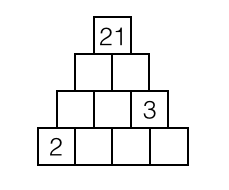You have to fill in all of the blank squares so that each square (other than those on the bottom) contains a number that is the sum of the two numbers in the squares below it. The solution to the above puzzle is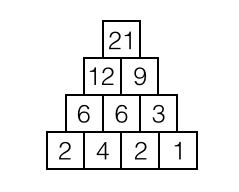Some puzzles have multiple solutions, and some have no solutions. Also, only positive integers are allowed.

I've come up with an interesting method of solving them... but before I tell you, try some puzzles! Here are a few good ones (I promise, they all have solutions).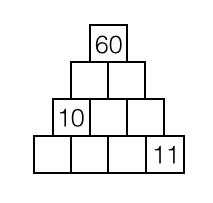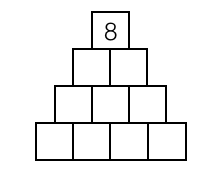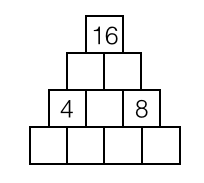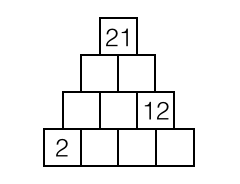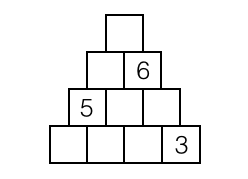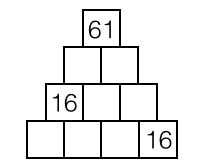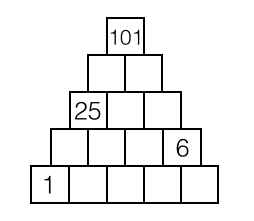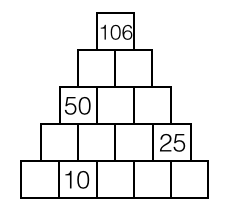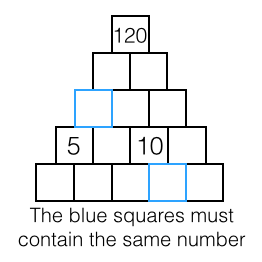Okay, now I'll explain my method of solving these puzzles. Using what we showed earlier, the number of paths from each square to the top of the pyramid is $_nC_r$, as long as we keep our definition of the location of a square. In a completed puzzle, each box contributes its value to each of the two boxes above it, and those boxes contribute its value up even further and so on - as if the value of each bottom box is being "passed up" to the top. At each split, the value that it contributes to the total amount in the topmost box is duplicated, meaning that the number of times that it contributes its value to the top is equal to the number of paths to the top, or $_nC_r$. Let us use this to solve this puzzle: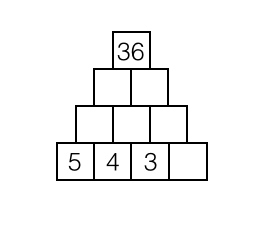We can start by assigning a variable $a$ to the blank box on the bottom: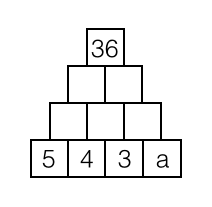Now we know that the weighted values of the bottom entries will sum to $36$, the topmost entry, so we can set up an equation to solve for $a$:

And sure enough, when we fill out the pyramid with $a=10$, it all works out perfectly. How satisfying.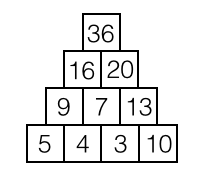Let's try a harder example:Our aim is to fill up the entire bottom row with values. However, this time we can't do it with just one variable. We have to use two: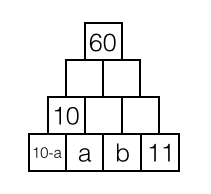Now we can set up an equation. This time, we will have a diophantine equation with two variables, and possibly more than one solution.

Remember that we are looking only for nonnegative integers. Not only that, but $10-a$ must also be nonnegative, so $a \lt 10$.

We have just reduced this to a simple diophantine equation. I'll save you the arithmetic. The possible values of $a$ and $b$, given by ordered pairs $(a,b)$, are as follows:

And so the three solutions to the puzzle are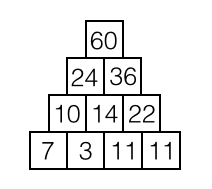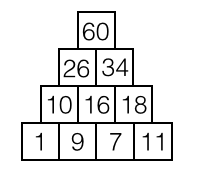The other puzzles can be solved in similar ways, but sometimes more algebra is required.

One last interesting thing about Pascal's Triangle is that the infinite sums of the reciprocals of the diagonals add up to nice, neat values. Of course, the first diagonal would be

which obviously diverges, and the second diagonal would be

which is the harmonic series, and is widely known to diverge. The sum of the second diagonal, however, is given by

and the third diagonal is

and the fourth is

We can quickly conjecture that the sum of the reciprocals of the entries of the nth diagonal is

However, proving such a statement is a trickier affair. I ended up proving it almost by accident. First let me establish the following identity:

I will omit the proof of this identity, as it is a purely algebraic proof and can be easily verified. Now let me show you how I accidentally stumbled upon an easy proof of our conjecture. I had set the function $S(x)$ equal to

in order to begin algebraically manipulating the equation and hopefully product a telescoping sum. I started by using the identity that I previously established

to split the sum into two disjoint sums, like this:

I then noticed that the sum on the left is identical to the sum $S(n)$ that we started with, so we have

And we subtract $S(n)$ from both sides to get

So, whilst trying to find a closed-form formula for $S(n)$, I accidentally found one for a related sum. This sum, though acquired accidentally, can actually be used to quickly prove the truth of our original conjecture. If we let $d(n)$ be

We can multiply both sides by $(x+1)$ to get

When we expand the $_iC_n$ into its full formula, something beautiful happens:

And finially, we substitute in for $d(n-1)$ to get

And there it is. Well, that about wraps up all of my shenanigans regarding Pascal's Triangle.